# Integration of Irrational Functions JEE Notes | EduRev

## JEE : Integration of Irrational Functions JEE Notes | EduRev

The document Integration of Irrational Functions JEE Notes | EduRev is a part of the JEE Course Mathematics (Maths) Class 12.
All you need of JEE at this link: JEE

Integration of Irrational Functions

Certain types of integrals of algebraic irrational expressions can be reduced to integrals of rational functions by a appropriate change of the variable. Such transformation of an integral is called its rationalization.

(i) If the integrand is a rational function of fractional powers of an independent variable x, i.e. the function R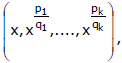then the integral can be rationalized by the substitution x = tm, where m is the least common multiple of the numbers q1, q2, ...., qk.

(ii) If the integrand is a rational function of x and fractional powers of a linear fractional function of the form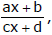then rationalization of the integral is effected by the substitution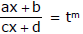where m has the same sense as above.

Ex.68 Evaluate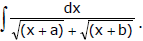Sol.

Rationalizing the denominator, we have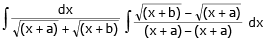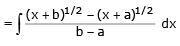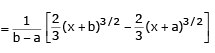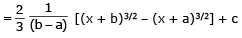Ex.69 Evaluate I =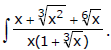Sol. The least common multiple of the numbers 3 and 6 is 6, therefore we make the substitution

x = t6, dx = 6t5 dt.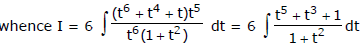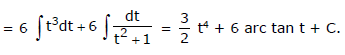Ex.70 Evaluate I =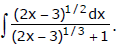Sol. The integrand is a rational function of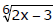therefore we put 2x – 3 = t6, whence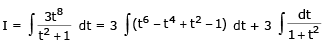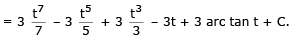Returning to x, we get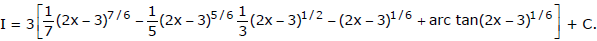Ex.71 Evaluate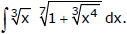Sol.

Let x = t3 ⇒ dx = 3t2 then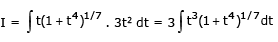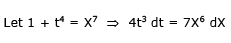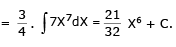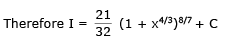Ex.72 Evaluate I =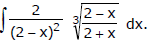Sol. The integrand is a rational function of x and the expression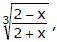therefore let us introduce the substitution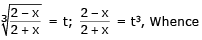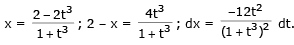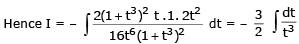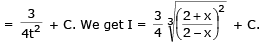INTEGRAL OF THE TYPE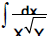WHERE X AND Y ARE LINEAR OR QUADRATIC EXPRESSION

Ex.73 Integrate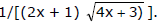Sol.

Put 4x + 3 = t2, so that 4dx = 2tdt and (2x + 1)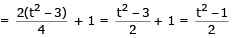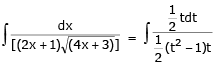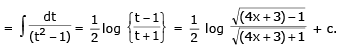Ex.74 Evaluate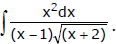Sol.

Put (x + 2) = t2, so that dx = 2t dt, Also x = t– 2.

∴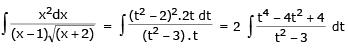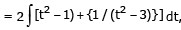dividing the numerator by the denominator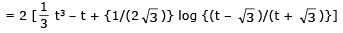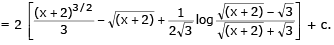Ex.75 Integrate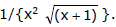Sol.

Put (x + 1) = t2, so that dx = 2t dt. Also x = t2 – 1.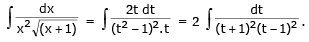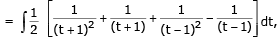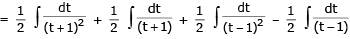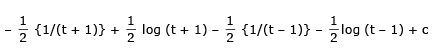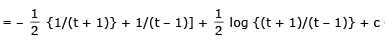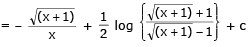Ex.76 Integrate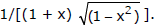Sol.

Put (1 + x) = 1/t, so that dx = – (1/t2) dx.

Also x = (1/t) – 1.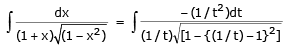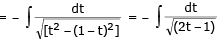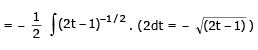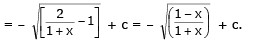Ex.77 Evaluate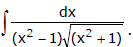Sol.

Put x = 1/t, so that dx = – (1/t2) dt.

∴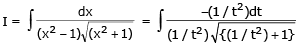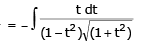Now put 1 + t2 = z2 so that t dt = z dz. Then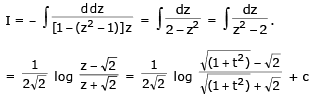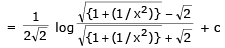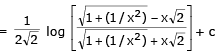[∵ t = 1/x]

Ex.78 Evaluate I =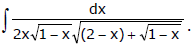Sol.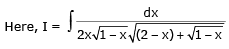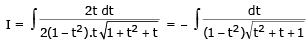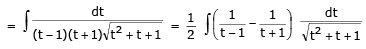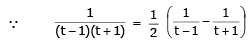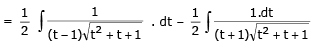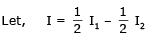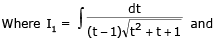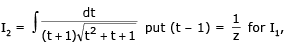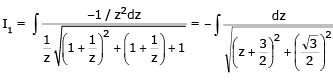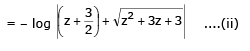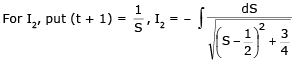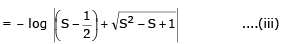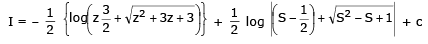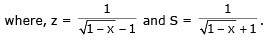Integration Of A Binomial Differential

The integral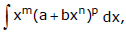where m, n, p are rational numbers, is expressed through elementary functions only in the following three cases :

Case I : p is an integer. Then, if p > 0, the integrand is expanded by the formula of the binomial; but if p < 0, then we put x = tk, where k is the common denominator of the fractions and n.

Case II :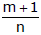is an integer. We put a + bxn = tα, where α is the denominator of the fraction p.

Case III :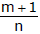+ p is an integer we put a + bxn = tαxn, where a is the denominator of the fraction p.

Ex.79 Evaluate I =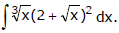Sol.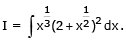Here p = 2, i.e. an integer, hence we have case I.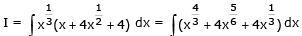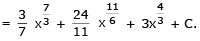Ex.80 Evaluate I =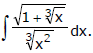Sol.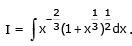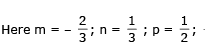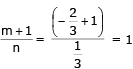i.e. an integer.we have case II. Let us make the substitution. Hence ,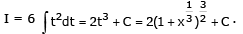Ex.81 Evaluate I =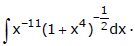Sol.

Here p = – 1/2 is a fraction, m+1/2 = -5/2 also a fraction, but m+1/n + p/2 = -5/2 -1/2 = -3 is an integer, i.e. we have case III, we put 1 + x4 = x4/2,

Hence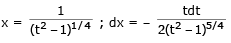Substituting these expression into the integral, we obtain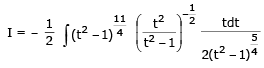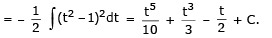Returning to x, we get I =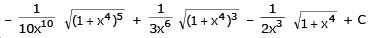Offer running on EduRev: Apply code STAYHOME200 to get INR 200 off on our premium plan EduRev Infinity!

## Mathematics (Maths) Class 12

209 videos|222 docs|124 tests

,

,

,

,

,

,

,

,

,

,

,

,

,

,

,

,

,

,

,

,

,

;